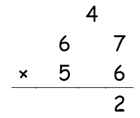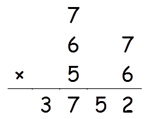This is method for multiplying two tow digit numbers. Let us say universally that the two numbers are AB and CD.

If you want to multiply them directly then following method is to be followed.

Write both numbers as shown below.

A  B
C  D

Step 1: B × D

Step 2: (A × D) + (B × C)

Step 3: A × C

Let’s take an example.

67 × 56

Step 1:  7 × 6 = 42Step 2: (6×6) + (5×7) = 71 +4(carry) = 75Step 3: 6 × 5 = 30 + 7(carry) = 37